## Locating and Ordering Fractions and Mixed Numbers on the Number Line

### Learning Outcomes

• Locate and label improper and proper fractions on a number line
• Order fractions and mixed numbers on a number line
• Use inequality symbols to compare fractions and mixed numbers

Now we are ready to plot fractions on a number line. This will help us visualize fractions and understand their values.

Let us locate ${\Large\frac{1}{5},\frac{4}{5}},3,3{\Large\frac{1}{3},\frac{7}{4},\frac{9}{2}},5$, and ${\Large\frac{8}{3}}$ on the number line.

We will start with the whole numbers $3$ and $5$ because they are the easiest to plot.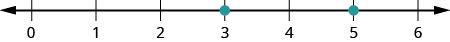The proper fractions listed are ${\Large\frac{1}{5}}$ and ${\Large\frac{4}{5}}$. We know proper fractions have values less than one, so ${\Large\frac{1}{5}}$ and ${\Large\frac{4}{5}}$ are located between the whole numbers $0$ and $1$. The denominators are both $5$, so we need to divide the segment of the number line between $0$ and $1$ into five equal parts. We can do this by drawing four equally spaced marks on the number line, which we can then label as ${\Large\frac{1}{5},\frac{2}{5},\frac{3}{5}}$, and ${\Large\frac{4}{5}}$.

Now plot points at ${\Large\frac{1}{5}}$ and ${\Large\frac{4}{5}}$.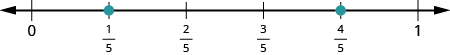The only mixed number to plot is $3{\Large\frac{1}{3}}$. Between what two whole numbers is $3{\Large\frac{1}{3}}$? Remember that a mixed number is a whole number plus a proper fraction, so $3{\Large\frac{1}{3}}>3$. Since it is greater than $3$, but not a whole unit greater, $3{\Large\frac{1}{3}}$ is between $3$ and $4$. We need to divide the portion of the number line between $3$ and $4$ into three equal pieces (thirds) and plot $3{\Large\frac{1}{3}}$ at the first mark.Finally, look at the improper fractions ${\Large\frac{7}{4},\frac{9}{2}}$, and ${\Large\frac{8}{3}}$. Locating these points will be easier if you change each of them to a mixed number.

${\Large\frac{7}{4}}=1{\Large\frac{3}{4}},{\Large\frac{9}{2}}=4{\Large\frac{1}{2}},{\Large\frac{8}{3}}=2{\Large\frac{2}{3}}$

Here is the number line with all the points plotted.### Example

Locate and label the following on a number line: ${\Large\frac{3}{4},\frac{4}{3},\frac{5}{3}},4{\Large\frac{1}{5}}$, and ${\Large\frac{7}{2}}$.

Solution:
Start by locating the proper fraction ${\Large\frac{3}{4}}$. It is between $0$ and $1$. To do this, divide the distance between $0$ and $1$ into four equal parts. Then plot ${\Large\frac{3}{4}}$.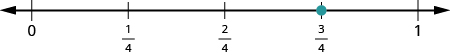Next, locate the mixed number $4{\Large\frac{1}{5}}$. It is between $4$ and $5$ on the number line. Divide the number line between $4$ and $5$ into five equal parts, and then plot $4{\Large\frac{1}{5}}$ one-fifth of the way between $4$ and $5$ .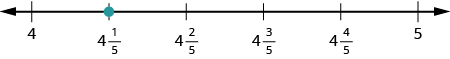Now locate the improper fractions ${\Large\frac{4}{3}}$ and ${\Large\frac{5}{3}}$. It is easier to plot them if we convert them to mixed numbers first.

${\Large\frac{4}{3}}=1{\Large\frac{1}{3}},{\Large\frac{5}{3}}=1{\Large\frac{2}{3}}$
Divide the distance between $1$ and $2$ into thirds.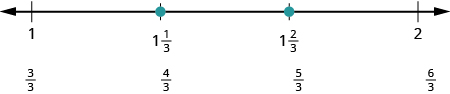Next let us plot ${\Large\frac{7}{2}}$. We write it as a mixed number, ${\Large\frac{7}{2}}=3{\Large\frac{1}{2}}$ . Plot it between $3$ and $4$.The number line shows all the numbers located on the number line.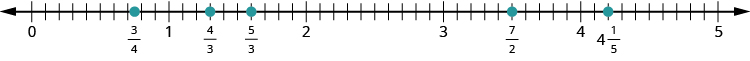### try it

Watch the following video to see more examples of how to locate fractions on a number line.

In Introduction to Integers, we defined the opposite of a number. It is the number that is the same distance from zero on the number line but on the opposite side of zero. We saw, for example, that the opposite of $7$ is $-7$ and the opposite of $-7$ is $7$.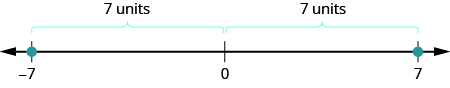Fractions have opposites, too. The opposite of ${\Large\frac{3}{4}}$ is $-{\Large\frac{3}{4}}$. It is the same distance from $0$ on the number line, but on the opposite side of $0$.Thinking of negative fractions as the opposite of positive fractions will help us locate them on the number line. To locate $-{\Large\frac{15}{8}}$ on the number line, first think of where ${\Large\frac{15}{8}}$ is located. It is an improper fraction, so we first convert it to the mixed number $1{\Large\frac{7}{8}}$ and see that it will be between $1$ and $2$ on the number line. So its opposite, $-{\Large\frac{15}{8}}$, will be between $-1$ and $-2$ on the number line.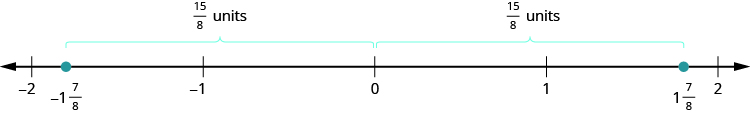### Example

Locate and label the following on the number line: ${\Large\frac{1}{4}},-{\Large\frac{1}{4}},1{\Large\frac{1}{3}},-1{\Large\frac{1}{3}},{\Large\frac{5}{2}}$, and $-{\Large\frac{5}{2}}$.

### Try it

In the next video we give more examples of how to locate negative and positive fractions on a number line.

## Order Fractions and Mixed Numbers

We can use the inequality symbols to order fractions. Remember that $a>b$ means that $a$ is to the right of $b$ on the number line. As we move from left to right on a number line, the values increase.

### Example

Order each of the following pairs of numbers, using $<$; or $>:$

1. $-{\Large\frac{2}{3}}$ ____ $- 1$
2. $-3{\Large\frac{1}{2}}$ ____ $- 3$
3. $-{\Large\frac{3}{7}}$ ____ ${\Large\frac{3}{8}}$
4. $-2$ ____ ${\Large\frac{-16}{9}}$

### Try it

In the following video we show another example of how to order integers, fractions and mixed numbers using inequality symbols.